# Regression Methods

[Page 691 ( continued )]

The time series techniques of exponential smoothing and moving average relate a single variable being forecast (such as demand) to time . In contrast, regression is a forecasting technique that measures the relationship of one variable to one or more other variables . For example, if we know that something has caused product demand to behave in a certain way in the past, we might like to identify that relationship. If the same thing happens again in the future, we can then predict what demand will be. For example, there is a well-known relationship between increased demand in new housing and lower interest rates. Correspondingly, a whole myriad of building products and services display increased demand if new housing starts increase. Similarly, an increase in sales of DVD players results in an increase in demand for DVDs.

[Page 692]

The simplest form of regression is linear regression, which you will recall we used previously to develop a linear trend line for forecasting. In the following section we will show how to develop a regression model for variables related to items other than time.

#### Linear Regression

Simple linear regression relates one dependent variable to one independent variable in the form of a linear equation: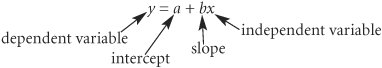Linear regression relates demand (dependent variable) to an independent variable .

To develop the linear equation, the slope, b , and the intercept, a , must first be computed by using the following least squares formulas: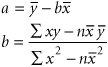where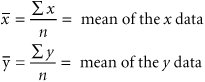We will consider regression within the context of an example. The State University athletic department wants to develop its budget for the coming year, using a forecast for football attendance. Football attendance accounts for the largest portion of its revenues , and the athletic director believes attendance is directly related to the number of wins by the team. The business manager has accumulated total annual attendance figures for the past 8 years :

Wins

Attendance

4

36,300

6

40,100

6

41,200

8

53,000

6

44,000

7

45,600

5

39,000

7

47,500

Given the number of returning starters and the strength of the schedule, the athletic director believes the team will win at least seven games next year. He wants to develop a simple regression equation for these data to forecast attendance for this level of success.

[Page 693]

The computations necessary to compute a and b , using the least squares formulas, are summarized in Table 15.10. (Note that the magnitude of y has been reduced to make manual computation easier.)

##### Table 15.10. Least squares computations

x (wins)

y (attendance, 1,000s)

xy

x 2

4

36.3

145.2

16

6

40.1

240.6

36

6

41.2

247.2

36

8

53.0

424.0

64

6

44.0

264.0

36

7

45.6

319.2

49

5

39.0

195.0

25

7

47.5

332.5

49

49

346.7

2,167.7

311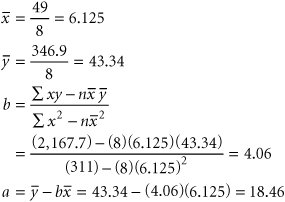Substituting these values for a and b into the linear equation line, we have

y = 18.46 + 4.06 x

Thus, for x = 7 (wins), the forecast for attendance is

y = 18.46 + 4.06(7) = 46.88 or 46,880

The data points with the regression line are shown in Figure 15.6. Observing the regression line relative to the data points, it would appear that the data follow a distinct upward linear trend, which would indicate that the forecast should be relatively accurate. In fact, the MAD value for this forecasting model is 1.41, which suggests an accurate forecast.

#### Correlation

Correlation in a linear regression equation is a measure of the strength of the relationship between the independent and dependent variables. The formula for the correlation coefficient is

Correlation is a measure of the strength of the relationship between independent and dependent variables .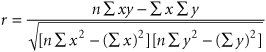The value of r varies between 1.00 and +1.00, with a value of ±1.00 indicating a strong linear relationship between the variables. If r = 1.00, then an increase in the independent variable will result in a corresponding linear increase in the dependent variable. If r = 1.00, an increase in the dependent variable will result in a linear decrease in the dependent variable. A value of r near zero implies that there is little or no linear relationship between variables.

[Page 694]
##### Figure 15.6. Linear regression line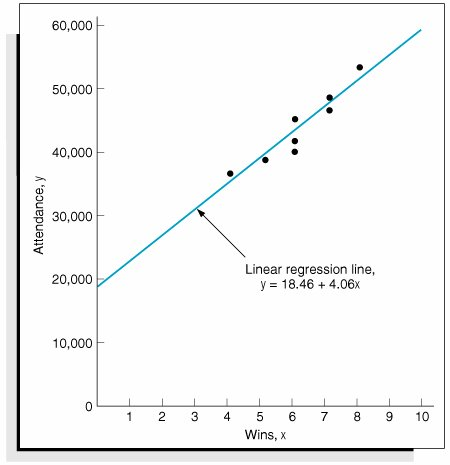We can determine the correlation coefficient for the linear regression equation determined in our State University example by substituting most of the terms calculated for the least squares formula (except for S y 2 ) into the formula for r :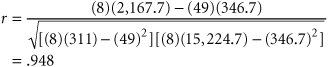This value for the correlation coefficient is very close to one, indicating a strong linear relationship between the number of wins and home attendance.

Another measure of the strength of the relationship between the variables in a linear regression equation is the coefficient of determination . It is computed by simply squaring the value of r . It indicates the percentage of the variation in the dependent variable that is a result of the behavior of the independent variable. For our example, r = .948; thus, the coefficient of determination is

The coefficient of determination is the percentage of the variation in the dependent variable that results from the independent variable .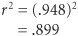This value for the coefficient of determination means that 89.9% of the amount of variation in attendance can be attributed to the number of wins by the team (with the remaining 10.1% due to other unexplained factors, such as weather, a good or poor start, publicity, etc.). A value of one (or 100%) would indicate that attendance totally depends on wins. However, because 10.1% of the variation is a result of other factors, some amount of forecast error can be expected.

[Page 695]

## Management Science Application: Forecasting Daily Demand in the Gas Industry

Vermont Gas Systems is a natural gas utility that serves approximately 26,000 business, industrial, and residential customers in 13 towns and cities in northwestern Vermont. Demand forecasts are a critical part of Vermont Gas Systems's supply chain that stretches across Canada from suppliers in western Canada to storage facilities along the TransCanada pipeline to Vermont Gas Systems's pipeline. Gas orders must be specified to suppliers at least 24 hours in advance. Vermont Gas Systems has storage capacity available for a buffer inventory of only 1 hour of gas use, so an accurate daily forecast of gas demand is essential.

Vermont Gas Systems uses regression to forecast daily gas demand. In its forecast models, gas demand is the dependent variable, and factors such as weather information and industrial customer demand are independent variables. During the winter, customers use more gas for heat, making an accurate weather forecast a very important factor. Detailed 3-day weather forecasts are provided to Vermont Gas Systems five times per day from a weather forecasting service. Individual regression forecasts are developed for 24 large-use industrial and municipal customers, such as factories, hospitals , and schools . End-use demand is the total potential capacity of all natural gas appliances in the system. It changes daily, as new customers move into a new house, apartment, or business, adding new appliances or equipment to the system. The utility uses only the most recent 30 days of demand data in developing its forecast models, and it updates the models on a weekly basis. Vermont Gas Systems interprets the results of the forecast model and supplements them with its individual knowledge of the supply chain distribution system and customer usage to develop an overall, accurate daily forecast of gas demand.

Columbia Gas Company in Ohio, a subsidiary of Virginia-based Columbia Energy Group , is the largest natural gas utility in Ohio, with nearly 1.3 million customers in more than 1,000 communities. Columbia employs two types of daily forecast: the design day forecast and the daily operational forecast. The design day forecast is used to determine the amount of gas supply, transportation capacity, and storage capacity that Columbia requires to meet its customer needs. It is very important that the design day forecast be accurate; if it is not, Columbia may not contract for enough gas from its suppliers, which could create shortages and put its customers at risk. The daily operational forecast is used to ensure that scheduled supplies are balanced with forecasted demands over the next 5-day period. It is used to balance supply and demand on a daily basis. The forecasting process is similar for the two types of forecasts. Columbia uses multiple regression analysis, based on daily demand for 2 years, and several weather-related independent variables to develop the parameters of a time series forecast model for the design day forecast and the daily operational forecast.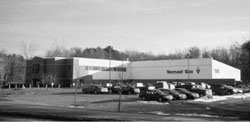Source: M. Flock, "Forecasting Winter Daily Gas Demand at Vermont Gas Systems," Journal of Business Forecasting 13, no. 1 (Spring 1994): 2; and H. Catron, "Daily Demand Forecasting at Columbia Gas," Journal of Business Forecasting 19, no. 2 (Summer 2000): 105.

#### Regression Analysis with Excel

Exhibit 15.8 shows a spreadsheet set up to develop the linear regression forecast for our State University athletic department example. Notice that Excel computes the slope directly with the formula = SLOPE(B5:B12, A5:A12) entered in cell E7 and shown on the formula bar at the top of the spreadsheet. The formula for the intercept in cell E6 is = INTERCEPT(B5:B12,A5:A12) . The values for the slope and intercept are subsequently entered in cells E9 and G9 to form the linear regression equation. The correlation coefficient in cell E13 is computed by using the formula = CORREL(B5:B12,A5:A12) . Although it is not shown on the spreadsheet, the coefficient of determination ( r 2 ) could be computed by using the formula = RSQ(B5:B12,A5:A12) .

##### (This item is displayed on page 696 in the print version)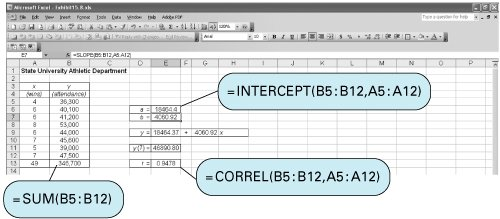The same linear regression equation could be computed in Excel if we had developed and entered the mathematical formulas for computing the slope and intercept we developed in the previous section, although that would have been more time-consuming and tedious .

[Page 696]

It is also possible to develop a scatter diagram of our example data similar to the chart shown in Figure 15.6 by using the Chart Wizard in Excel. First, cover the example data in cells A5:B12 on the spreadsheet in Exhibit 15.8. Next click on "Insert" on the toolbar at the top of the spreadsheet. This will result in the menu shown in Exhibit 15.9.

##### Exhibit 15.9.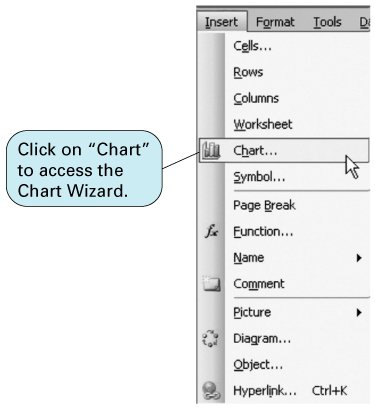Select "Chart" from this menu, which will access the Chart Wizard window. In the Chart Wizard window select the "XY (Scatter)" chart from the "Chart Type" menu, as shown in Exhibit 15.10.

Clicking on "Next" on the window in Exhibit 15.10 will provide a preliminary plot of the example data. (If you forgot to cover your example data cells earlier, you will be asked to do so at this point; this is the range A5:B12 .) Clicking on "Next" will enable you to add or delete chart legends, title the chart and the axes, and generally customize your chart. Clicking on "Finish" will display the chart on your spreadsheet so that you can position it, reduce it, expand it, or work on it some more. Exhibit 15.11 shows our spreadsheet with the scatter diagram chart for our example data.

[Page 697]
##### Exhibit 15.10.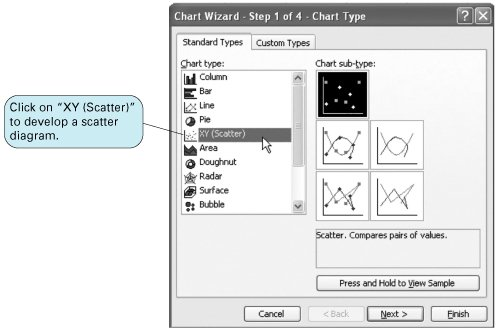##### Exhibit 15.11.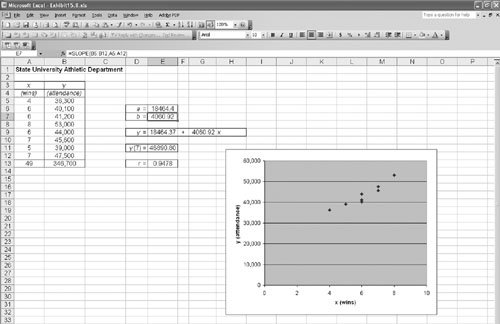[Page 698]

A linear regression forecast can also be developed directly with Excel by using the "Data Analysis" option from the "Tools" menu we accessed previously to develop an exponentially smoothed forecast. Exhibit 15.12 shows the "Regression" selection from the Data Analysis window and Exhibit 15.13 shows the Regression window. We first enter the cells from Exhibit 15.8 that include the y values (for attendance), B5:B12 . Next, we enter the x value cells, A5:A12 . The output range is the location on the spreadsheet where you want to put the output results. This range needs to be large (18 cells by 9 cells) and must not overlap with anything else on the spreadsheet. Clicking on "OK" will result in the spreadsheet shown in Exhibit 15.14. (Note that the "Summary Output" section has been slightly editedi.e., moved aroundso that all the results could be included on the screen in Exhibit 15.14.)

##### Exhibit 15.12.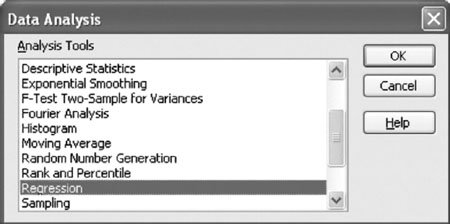##### Exhibit 15.13.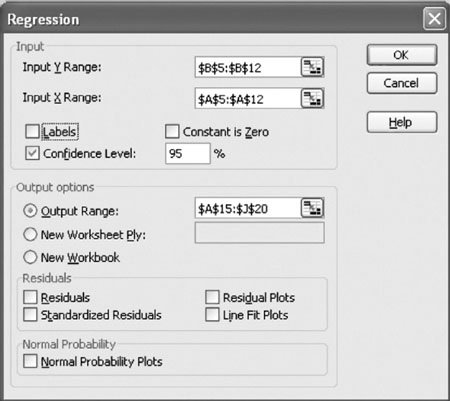##### (This item is displayed on page 699 in the print version)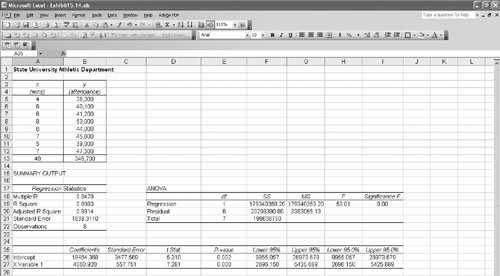The "Summary Output" section in Exhibit 15.14 provides a large amount of statistical information, the explanation and use of which are beyond the scope of this text. The essential items that interest us are the intercept and slope (labeled "X Variable 1") in the "Coefficients" column at the bottom of the spreadsheet and the "Multiple R" (or correlation coefficient) value shown under "Regression Statistics."

[Page 699]

Note the Excel QM also has a spreadsheet macro for regression analysis that can be accessed similarly to the exponentially smoothed forecast in Exhibit 15.15.

##### Exhibit 15.15.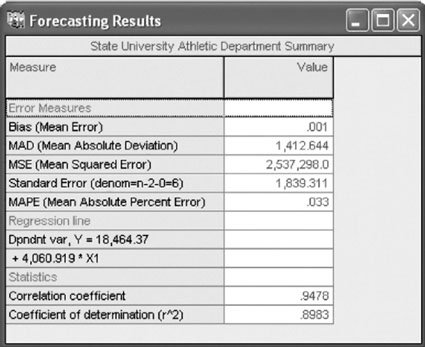#### Regression Analysis with QM for Windows

QM for Windows has the capability to perform linear regression, as demonstrated earlier. To demonstrate this program module, we will use our State University athletic department example. The program output, including the linear equation and correlation coefficient, is shown in Exhibit 15.15.

[Page 700]

#### Multiple Regression with Excel

Another causal method of forecasting is multiple regression , a more powerful extension of linear regression. Linear regression relates a dependent variable such as demand to one other independent variable, whereas multiple regression reflects the relationship between a dependent variable and two or more independent variables. A multiple regression model has the following general form:

y = b + b 1 x 1 + b 2 x 2 + . . . + b k x k

where

 b = the intercept b 1 . . . b k = parameters representing the contribution of the independent variables x 1 . . . x k = independent variables

Multiple regression relates demand to two or more independent variables .

For example, the demand for new housing ( y ) in a region or an urban area might be a function of several independent variables, including interest rates, population, housing prices, and personal income. Development and computation of the multiple regression equation, including the compilation of data, are quite a bit more complex than linear regression. Therefore, the only viable means for forecasting using multiple regression problems is by using a computer.

To demonstrate the capability to solve multiple regression problems with Excel spreadsheets, we will expand our State University athletic department example for forecasting attendance at football games that we used to demonstrate linear regression. Instead of attempting to predict attendance based on only one variable (wins), we will include a second variable for advertising and promotional expenditures, as follows :

Wins

Promotion

Attendance

4

\$29,500

36,300

6

55,700

40,100

6

71,300

41,200

8

87,000

53,000

6

75,000

44,000

7

72,000

45,600

5

55,300

39,000

7

81,600

47,500

We will use the "Data Analysis" option (add-in) from the "Tools" menu at the top of the spreadsheet that we used in the previous section to develop our linear regression equation, and then we will use the "Regression" option from the "Data Analysis" menu. The resulting spreadsheet, with the multiple regression statistics, is shown in Exhibit 15.16.

##### (This item is displayed on page 701 in the print version)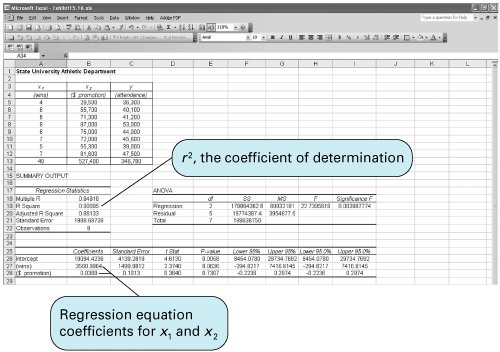Note that the data need to be set up on the spreadsheet so that the x variables are in adjacent columns (in this case, columns A and B). Then we enter the "Input x Range" as A4:B12 , as shown in Exhibit 15.17. Notice that we have also included cells A4, B4, and C4, which include our variable headings (i.e., "wins," "\$ promotion," and "attendance"), in the input ranges. By clicking on "Labels," headings can be placed on our spreadsheet in cells A27 and A28.

##### (This item is displayed on page 701 in the print version)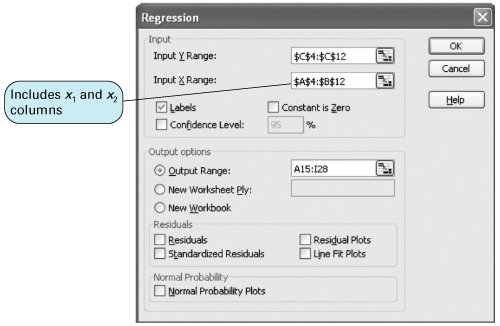The regression coefficients for our x variables, wins and promotion, are shown in cells B27 and B28 in Exhibit 15.16. Thus, the multiple regression equation is formulated as

y = 19,094.42 + 3,560.99 x 1 + .0368 x 2

[Page 701]

This equation can now be used to forecast attendance based on both projected football wins and promotional expenditure. For example, if the athletic department expects the team to win seven games and plans to spend \$60,000 on promotion and advertising, the forecasted attendance is

 y = 19,094.42 + 3,560.99(7) +.0368(60,000) = 46,229.35

[Page 702]

If the promotional expenditure is held constant, every win will increase attendance by 3,560.99, whereas if the wins are held constant, every \$1,000 of advertising money spent will increase attendance by 36.8 fans. This would seem to suggest that number of wins has a more significant impact on attendance than promotional expenditures.

The coefficient of determination, r 2 , shown in cell B19 in Exhibit 15.16, is .90, which suggests that 90% of the amount of variation in attendance can be attributed to the number of wins and the promotional expenditures. However, as we have already noted, the number of wins probably accounts for a larger part of the variation in attendance.

A problem often encountered in multiple regression is multicollinearity , or the amount of "overlapping" information about the dependent variable that is provided by several independent variables. This problem usually occurs when the independent variables are highly correlated, as in this example, in which wins and promotional expenditures are both positively correlated; that is, more wins coincide with higher promotional expenditures and vice versa. (Possibly the athletic department increased promotional expenditures when it thought it would have a better team that would achieve more wins.) Multicollinearity and how to cope with it are beyond the scope of this text and this brief section on multiple regression; however, most statistics texts discuss this topic in detail.Introduction to Management Science (10th Edition)
ISBN: 0136064361
EAN: 2147483647
Year: 2006
Pages: 358

Similar book on Amazon

flylib.com © 2008-2017.
If you may any questions please contact us: flylib@qtcs.net